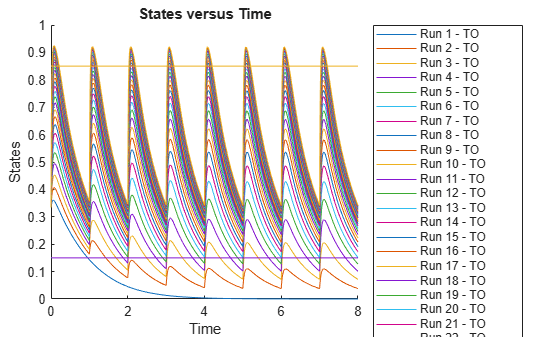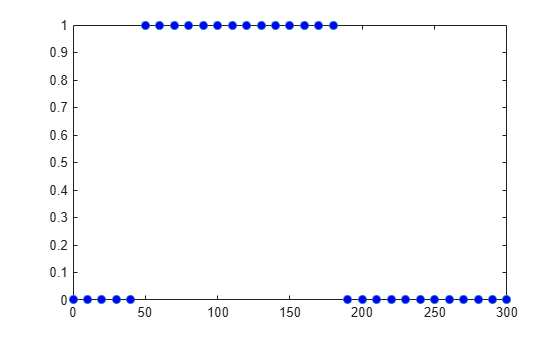Main Content

# Observable

Object containing expression for post-simulation calculations

## Description

An observable object is a mathematical expression that lets you perform post-simulation calculations. For example, you can define an observable expression to compute the fraction of a ligand that is bound to a receptor at each time step, or compute some statistics such as area under the curve (AUC) of a drug concentration profile. You can also use an observable object as a response in simulation, data fitting, and global sensitivity analysis.

The name of each observable object in a SimBiology® model must be unique, meaning no observable object can have the same name as another observable, species, compartment, parameter, reaction, variant, or dose in the model. An observable object can reference any model quantities that are logged (in `StatesToLog`). It can also reference other active observable objects provided that the expressions contain no algebraic loops. The object expression can reference simulation time using the variable time. Follow the recommended guidelines for expression evaluations. For instance, if a quantity name is not a valid MATLAB® variable name, enclose the name in brackets `[]` when referring to it in an expression.

SimBiology evaluates the object expression using the entire time course of any referenced states or observables. The result of an observable expression must be a numeric scalar or vector. If it is a vector, it must be of the same length as the simulation time vector. The result is stored in the returned `SimData` object. Specifically, if the observable expression is scalar-valued, the result is stored in the `SimData.ScalarObservables` property. Otherwise, it is stored in `SimData.VectorObservables`.

Note

• Make sure to correctly vectorize the expressions. For example, use `A./(A+B)` instead of `A/(A+B)` if A and B are matrices.

• Avoid hardcoding expressions that expect any particular number of points or times. For example, instead of using `time(1:1000)`, use `time(1:min(1000,numel(time)))`.

## Creation

Create an observable object using `addobservable`.

## Properties

expand all

Mathematical expression of the observable object, specified as a character vector.

Example: `'x.^2'`

Data Types: `char`

Units of the observable expression results, specified as a character vector.

Example: `'gram'`

Data Types: `char`

Flag indicating whether to evaluate the observable expression after model simulation, specified as `true` or `false`.

Example: `false`

Data Types: `logical`

Object name, specified as a character vector.

Example: `'AUC'`

Data Types: `char`

This property is read-only.

Parent object of the observable object, specified as a model object.

Description of the object, specified as a character vector.

Example: `'Drug AUC'`

Data Types: `char`

Object label, specified as a character vector.

Example: `'area under the curve'`

Data Types: `char`

This property is read-only.

Object type, specified as `'observable'`.

Data Types: `char`

Data to associate with the object, specified as any supported MATLAB data type.

## Object Functions

 `copyobj` Copy SimBiology object and its children `findUsages` Find out how observable object is used in SimBiology model `get` Get SimBiology object properties `set` Set SimBiology object properties `delete` Delete SimBiology object `display` Display summary of SimBiology object `rename` Rename object and update expressions

## Examples

collapse all

`sbioloadproject tmdd_with_TO.sbproj`

Set the target occupancy (`TO`) as a response.

```cs = getconfigset(m1); cs.RuntimeOptions.StatesToLog = 'TO';```

Get the dosing information.

`d = getdose(m1,'Daily Dose');`

Scan over different dose amounts using a `SimBiology.Scenarios` object. To do so, first parameterize the `Amount` property of the dose. Then vary the corresponding parameter value using the `Scenarios` object.

```amountParam = addparameter(m1,'AmountParam','Units',d.AmountUnits); d.Amount = 'AmountParam'; d.Active = 1; doseSamples = SimBiology.Scenarios('AmountParam',linspace(0,300,31));```

Create a `SimFunction` to simulate the model. Set `TO `as the simulation output.

```% Suppress informational warnings that are issued during simulation. warning('off','SimBiology:SimFunction:DOSES_NOT_EMPTY'); f = createSimFunction(m1,doseSamples,'TO',d)```
```f = SimFunction Parameters: Name Value Type Units _______________ _____ _____________ ____________ {'AmountParam'} 1 {'parameter'} {'nanomole'} Observables: Name Type Units ______ _____________ _________________ {'TO'} {'parameter'} {'dimensionless'} Dosed: TargetName TargetDimension Amount AmountValue AmountUnits _______________ ___________________________________ _______________ ___________ ____________ {'Plasma.Drug'} {'Amount (e.g., mole or molecule)'} {'AmountParam'} 1 {'nanomole'} ```
`warning('on','SimBiology:SimFunction:DOSES_NOT_EMPTY');`

Simulate the model using the dose amounts generated by the `Scenarios` object. In this case, the object generates 31 different doses; hence the model is simulated 31 times and generates a `SimData` array.

```doseTable = getTable(d); sd = f(doseSamples,cs.StopTime,doseTable)```
``` SimBiology Simulation Data Array: 31-by-1 ModelName: TMDD Logged Data: Species: 0 Compartment: 0 Parameter: 1 Sensitivity: 0 Observable: 0 ```

Plot the simulation results. Also add two reference lines that represent the safety and efficacy thresholds for `TO`. In this example, suppose that any `TO` value above 0.85 is unsafe, and any `TO` value below 0.15 has no efficacy.

```h = sbioplot(sd); time = sd(1).Time; h.NextPlot = 'add'; safetyThreshold = plot(h,[min(time), max(time)],[0.85, 0.85],'DisplayName','Safety Threshold'); efficacyThreshold = plot(h,[min(time), max(time)],[0.15, 0.15],'DisplayName','Efficacy Threshold');```Postprocess the simulation results. Find out which dose amounts are effective, corresponding to the `TO` responses within the safety and efficacy thresholds. To do so, add an observable expression to the simulation data.

```% Suppress informational warnings that are issued during simulation. warning('off','SimBiology:sbservices:SB_DIMANALYSISNOTDONE_MATLABFCN_UCON'); newSD = addobservable(sd,'stat1','max(TO) < 0.85 & min(TO) > 0.15','Units','dimensionless')```
``` SimBiology Simulation Data Array: 31-by-1 ModelName: TMDD Logged Data: Species: 0 Compartment: 0 Parameter: 1 Sensitivity: 0 Observable: 1 ```

The addobservable function evaluates the new observable expression for each `SimData` in `sd` and returns the evaluated results as a new `SimData` array, `newSD`, which now has the added observable (`stat1`).

SimBiology stores the observable results in two different properties of a `SimData` object. If the results are scalar-valued, they are stored in `SimData.ScalarObservables`. Otherwise, they are stored in `SimData.VectorObservables`. In this example, the `stat1` observable expression is scalar-valued.

Extract the scalar observable values and plot them against the dose amounts.

```scalarObs = vertcat(newSD.ScalarObservables); doseAmounts = generate(doseSamples); figure plot(doseAmounts.AmountParam,scalarObs.stat1,'o','MarkerFaceColor','b')```The plot shows that dose amounts ranging from 50 to 180 nanomoles provide `TO` responses that lie within the target efficacy and safety thresholds.

You can update the observable expression with different threshold amounts. The function recalculates the expression and returns the results in a new `SimData` object array.

`newSD2 = updateobservable(newSD,'stat1','max(TO) < 0.75 & min(TO) > 0.30');`

Rename the observable expression. The function renames the observable, updates any expressions that reference the renamed observable (if applicable), and returns the results in a new `SimData` object array.

`newSD3 = renameobservable(newSD2,'stat1','EffectiveDose');`

Restore the warning settings.

`warning('on','SimBiology:sbservices:SB_DIMANALYSISNOTDONE_MATLABFCN_UCON');`

## See Also

Introduced in R2020a

## SupportGet trial now